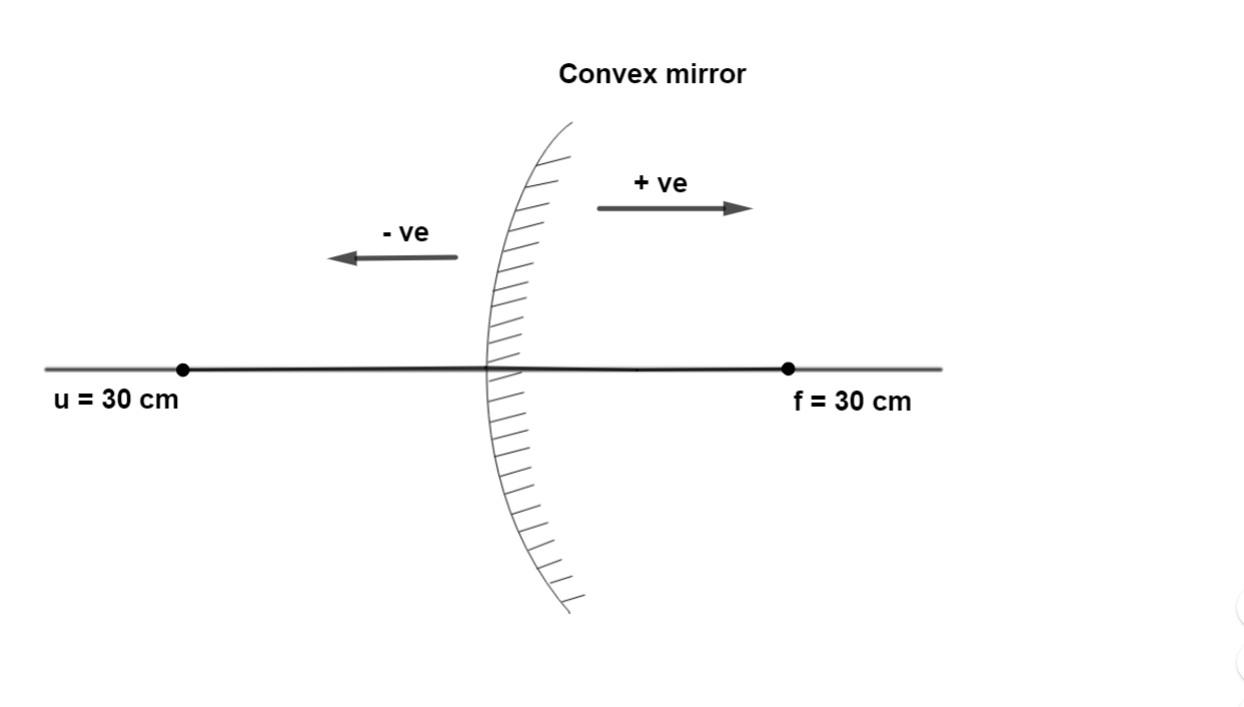Question

# A point object is placed at a distance of $30\mathrm{cm}$ from a convex mirror of focal length of $30\mathrm{cm}$. The image will form at________

Open in App
Solution

## Step 1. Given dataAccording to the sign convention in mirror, the object distance $\mathrm{u}=-30\mathrm{cm}$,Focal length $\mathrm{f}=30\mathrm{cm}$We have to find image distance $\mathrm{v}$.Step 2. Formula to be used.Mirror formula:A mirror can be made by silvering a metal surface with glass in front and paint at its back.The mirror formula represent the relation between focal length, image distance and object distance, $\frac{1}{\mathrm{f}}=\frac{1}{\mathrm{u}}+\frac{1}{\mathrm{v}}$Here, $\mathrm{f}$ is focal length, $\mathrm{v}$ is the distance of the image from the mirror and $\mathrm{u}$ is the distance of the object from the mirror.Step 3. Calculate the image distance.Now, by using the mirror formula, we get, $\frac{1}{30}=\frac{1}{-30}+\frac{1}{\mathrm{v}}$ $\frac{1}{\mathrm{v}}=\frac{1}{30}+\frac{1}{30}$ $\frac{1}{\mathrm{v}}=\frac{2}{30}$ $2\mathrm{v}=30$ $\mathrm{v}=15$The sign obtained is positive and the image distance is $15\mathrm{cm}$.According to the sign convention in mirror, the image is formed $15\mathrm{cm}$ behind the mirror.Hence, A point object is placed at a distance of $30\mathrm{cm}$ from a convex mirror of focal length of $30\mathrm{cm}$. The image will form at $15$ cm behind the image.Suggest Corrections6Similar questionsExplore more